Next: How can one enumerate Up: Convex Polyhedron Previous: What is the Minkowski-Weyl   Contents

## What is the vertex enumeration problem, and what is the facet enumeration problem?

When a polyhedron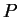in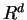has at least one extreme point and full dimensional, both representations (a) and (b) in Miknowski-Weyl Theorem 5 are unique up positive multiples of each inequality and ray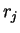.

Under these regularity conditions, the conversions between the H-representation and the V-representation are well-defined fundamental problems. The transformation (a) to (b) is known as the vertex enumeration and the other (b) to (a) is known as the facet enumeration. Whenis in addition bounded (i.e. polytope), the facet enumeration problem reduces to what we call the convex hull problem, see 2.10.

If a given polyhedron does not satisfy the assumptions, it is easy to transform the polyhedron to an isomorphic lower dimensional polyhedron satisfying the assumptions.

There are easy (nondegenerate) cases and difficult (degenerate) cases. For simplicity, we assume thatis bounded (i.e. polytope). The vertex enumeration is called nondegenerate if there is no point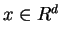which satisfies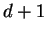given inequalities with equality, and degenerate otherwise. The facet enumeration is called nondegenerate if there is no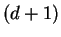given points which are on a common hyperplane, and degenerate otherwise.Next: How can one enumerate Up: Convex Polyhedron Previous: What is the Minkowski-Weyl   Contents
Komei Fukuda 2004-08-26